## Purpose

• test whether you have everything set up for the workshop
• retrieve and explore animal movement data from Movebank
• illustrate how to work with functions in the animal movement tools package (amt)
• write out available points for further annotating in EnvDATA

#### Preamble

library(knitr)
library(lubridate)
library(maptools)
library(raster)
library(move)
library(amt)
library(ggmap)
library(tibble)
library(leaflet)
library(dplyr)
options(width=165,digits.secs = 3)
lat = mean(fisherF2$location_lat)), zoom = 12, maptype = "hybrid", source = "google") ## Map from URL : http://maps.googleapis.com/maps/api/staticmap?center=42.751612,-73.902532&zoom=12&size=640x640&scale=2&maptype=hybrid&language=en-EN&sensor=false ggmap(map) + geom_point(data=fisherF2, aes(x=location_long, y=location_lat), size=2.5)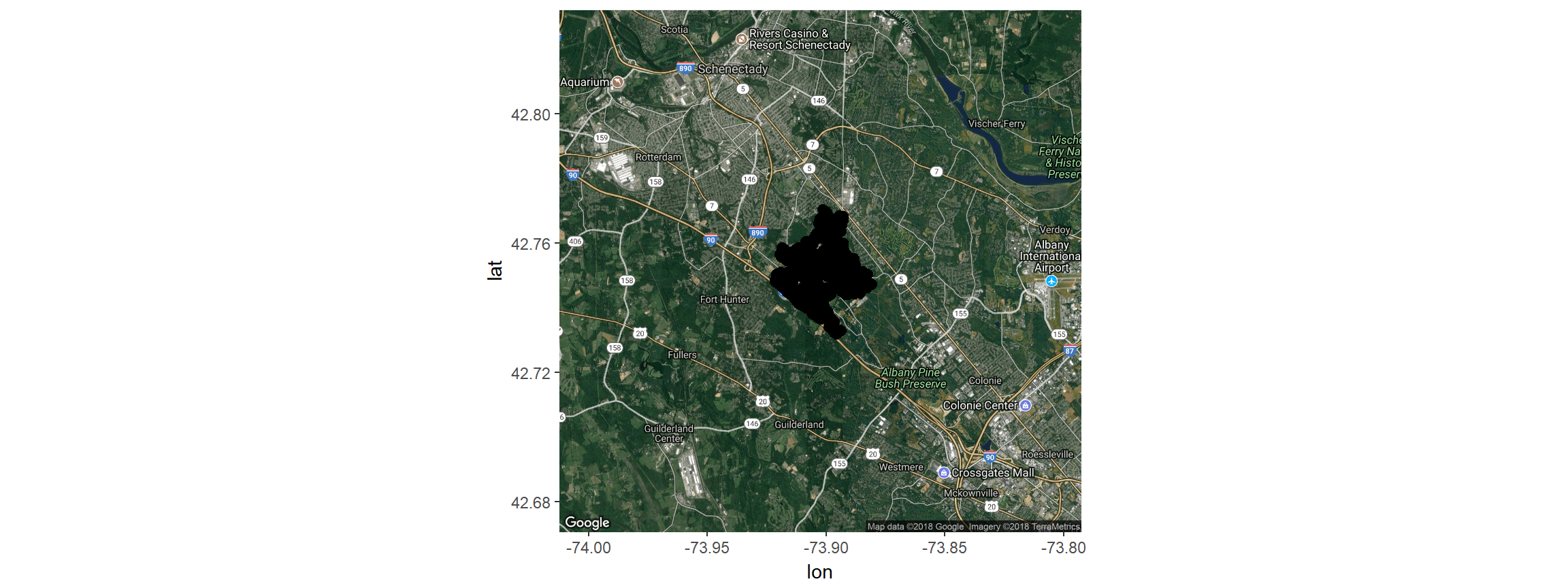# plot the track on the map Now, using leaflet leaflet(fisherF2)%>%addTiles()%>% addCircles(fisherF2$location_long, fisherF2$location_lat) ### Using ggplot without a background Use separate axes for each individual (add scales=“free” to facet_wrap) ggplot(fisher.dat, aes(x=location_long, y=location_lat))+geom_point()+ facet_wrap(~local_identifier, scales="free")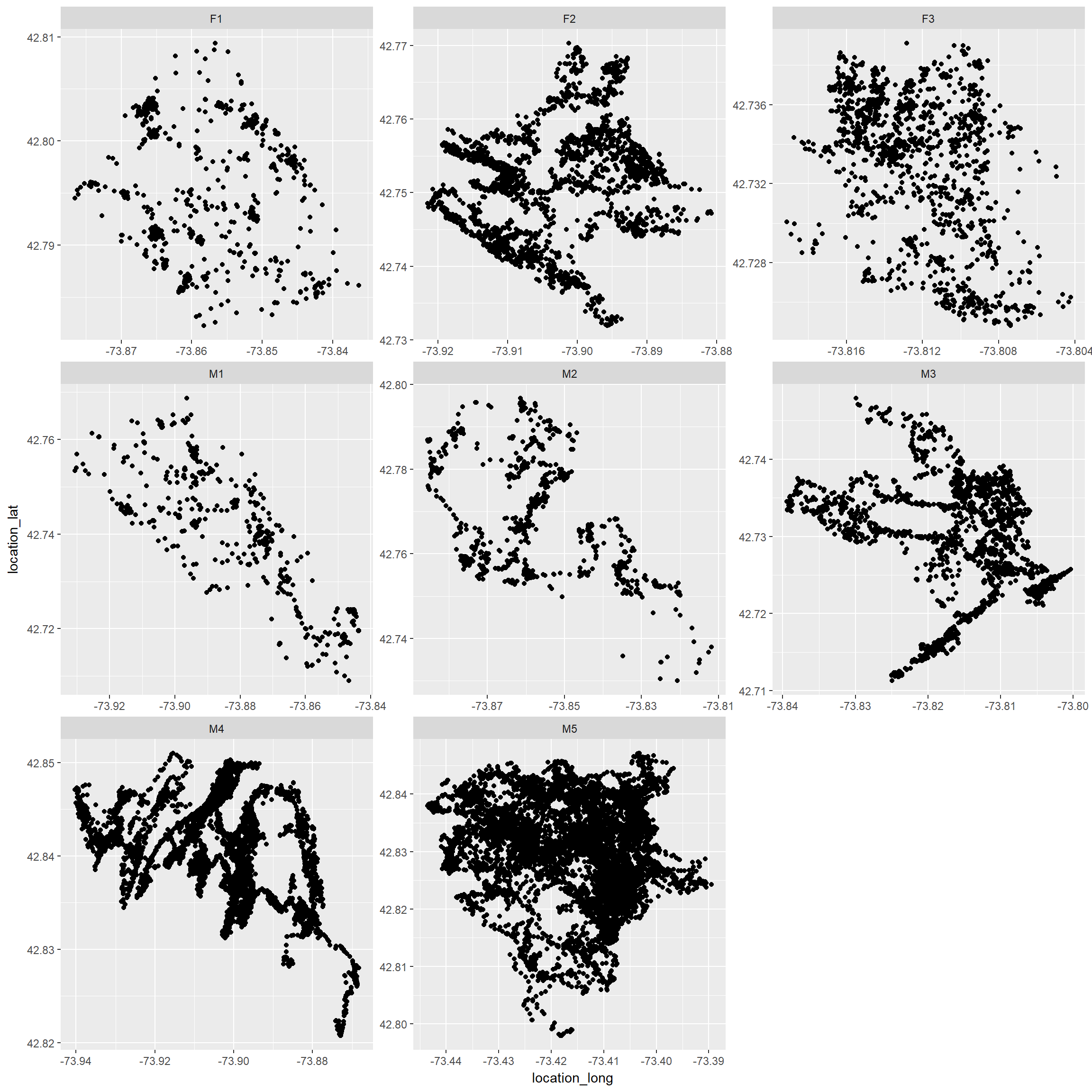Now, all on 1 plot ggplot(fisher.dat, aes(x=location_long, y=location_lat, color=as.factor(local_identifier)))+ geom_point()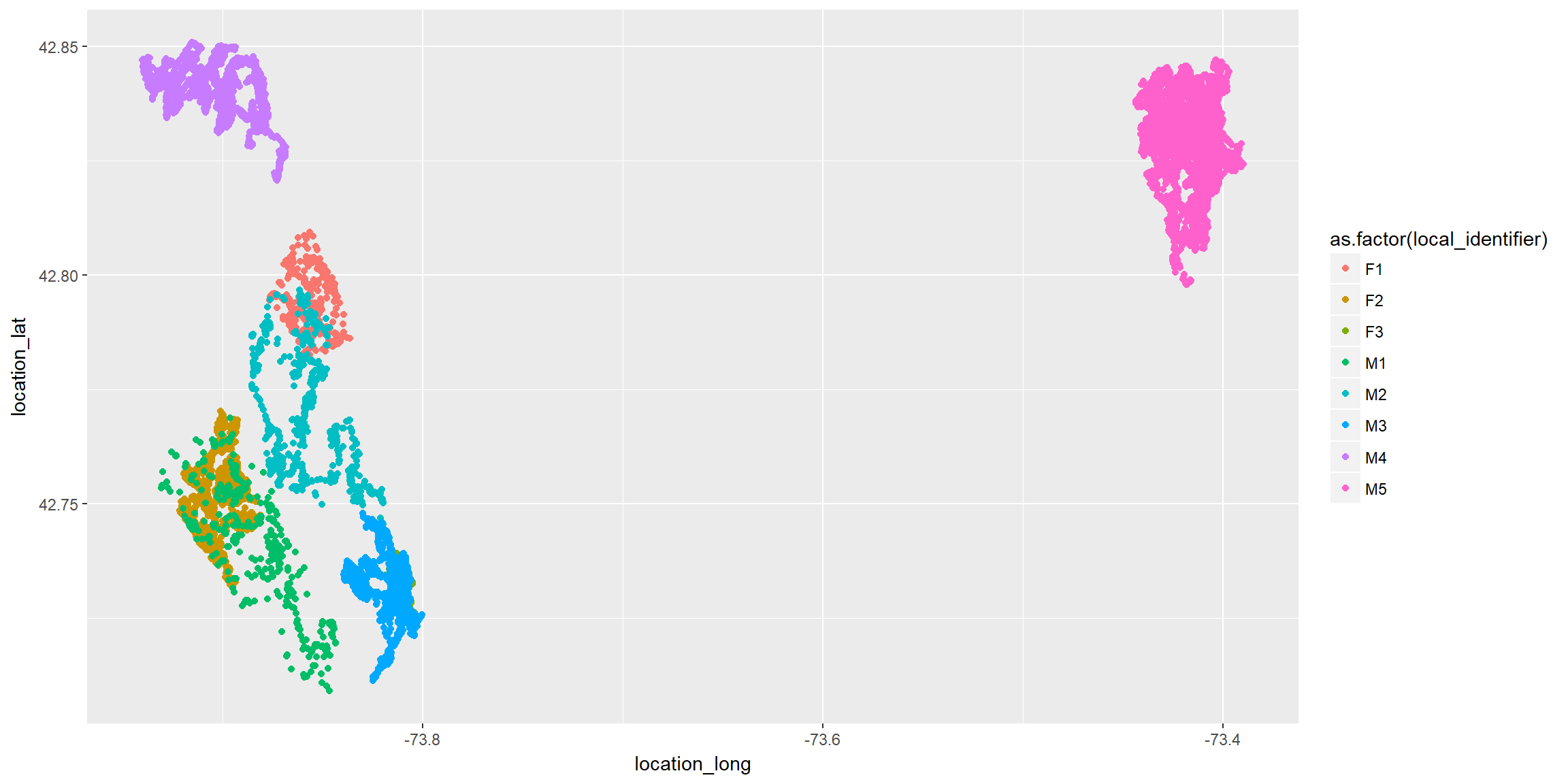## Creating a track in amt Before we can use the amt package to calculate step lengths, turn angles, and bearings for fisher data, we need to add a class (track) to the data. Then, we can summarize the data by individual, month, etc. First, create a track using utms and the timestamp. If we have a data set with locations in utms, we could use: #trk.temp <- make_track(fisher.dat, .x=utm.easting, .y=utm.northing, .t=timestamp, id = individual_local.identifier) #trk.temp Note: we did not need to explicitly specify x, y and t (but probably good to do so). This would also have worked trk <- make_track(fisher.dat, utm.easting, utm.northing, timestamp, id = local_identifier) We can also use lat, long, which will allow us to determine time of day trk <- mk_track(fisher.dat, .x=location_long, .y=location_lat, .t=timestamp, id = local_identifier, crs = CRS("+init=epsg:4326")) ## .t found, creating track_xyt. # Now it is easy to calculate day/night with either movement track trk <- trk %>% time_of_day() Now, we can transform back to geographic coordinates trk <- transform_coords(trk, CRS("+init=epsg:32618")) If we create trk.temp as above, we can make sure that we got back the same coordinates by comparing to the original track #mean(trk$x_ - trk.temp$x_) # look good, there is virtually no difference #mean(trk$y_ - trk.temp$y_) # same for y Save the class here (and apply it later after adding columns to the object) trk.class<-class(trk) ## Movement Characteristics • dir_abs will calculate absolute angles for steps • dir_rel will calculate turning angles (relative angles) • step_lengths will calculate distances between points • nsd = Net Squared Displacement (distance from first point) Arguments direction_abs: • full_circle will calculate between 0 and 360 (rather than -180 and 180) • zero gives the direction = 0 for absolute angle Note: we have to calculate these characteristics separately for each individual (to avoid calculating a distance between say the last observation of the first individual and the first observation of the second individual). To do this, we could loop through individuals, calculate these characteristics for each individual, then rbind the data back together. Or, use nested data frames and the map function in the purrr library to do this with very little code. To see how nesting works, we can create a nested object by individual nesttrk<-trk%>%nest(-id) nesttrk ## # A tibble: 8 x 2 ## id data ## <fct> <list> ## 1 M1 <tibble [919 x 4]> ## 2 M4 <tibble [8,958 x 4]> ## 3 F2 <tibble [3,004 x 4]> ## 4 M3 <tibble [2,436 x 4]> ## 5 F3 <tibble [1,501 x 4]> ## 6 M2 <tibble [1,638 x 4]> ## 7 F1 <tibble [1,349 x 4]> ## 8 M5 <tibble [13,099 x 4]> Each row contains data from an individual. For example, we can access data from the first individual using: nesttrk$data[]
## # A tibble: 919 x 4
##         x_       y_ t_                      tod_
##  *   <dbl>    <dbl> <dttm>                  <chr>
##  1 590130. 4732942. 2009-02-11 12:16:45.000 day
##  2 590136. 4732940. 2009-02-11 12:31:38.000 day
##  3 590138. 4732935. 2009-02-11 12:45:48.997 day
##  4 590144. 4732947. 2009-02-11 13:00:16.002 day
##  5 590137. 4732940. 2009-02-11 13:15:19.000 day
##  6 590126. 4732936. 2009-02-11 13:30:13.000 day
##  7 590139. 4732941. 2009-02-11 13:45:37.000 day
##  8 590139. 4732923. 2009-02-11 14:00:35.000 day
##  9 590137. 4732934. 2009-02-11 14:15:49.000 day
## 10 590136. 4732935. 2009-02-11 14:30:49.000 day
## # ... with 909 more rows

We could calculate movement characteristics by individual using:

temp<-direction_rel(nesttrk$data[]) head(temp) ##  NA -55.96892 130.93489 159.69985 -24.84488 179.16947 or: temp<-trk %>% filter(id=="M1") %>% direction_rel head(temp) ##  NA -55.96892 130.93489 159.69985 -24.84488 179.16947 Or, we can add a columns to each nested column of data using purrr::map trk<-trk %>% nest(-id) %>% mutate(dir_abs = map(data, direction_abs,full_circle=TRUE, zero="N"), dir_rel = map(data, direction_rel), sl = map(data, step_lengths), nsd_=map(data, nsd))%>%unnest() Now, calculate month, year, hour, week of each observation and append these to the dataset Unlike the movement charactersitics, these calculations can be done all at once, since they do not utilize successive observations (like step lengths and turn angles do). trk<-trk%>% mutate( week=week(t_), month = month(t_, label=TRUE), year=year(t_), hour = hour(t_) ) Now, we need to again tell R that this is a track (rather than just a data frame) class(trk) ##  "tbl_df" "tbl" "data.frame" class(trk)<-trk.class Lets take a look at what we created trk ## # A tibble: 32,904 x 13 ## id dir_abs dir_rel sl nsd_ x_ y_ t_ tod_ week month year hour ## <fct> <dbl> <dbl> <dbl> <dbl> <dbl> <dbl> <dttm> <chr> <dbl> <ord> <dbl> <int> ## 1 M1 259. NA 6.38 0 590130. 4732942. 2009-02-11 12:16:45.000 day 6 Feb 2009 12 ## 2 M1 203. -56.0 5.61 40.7 590136. 4732940. 2009-02-11 12:31:38.000 day 6 Feb 2009 12 ## 3 M1 334. 131. 12.7 112. 590138. 4732935. 2009-02-11 12:45:48.997 day 6 Feb 2009 12 ## 4 M1 134. 160. 9.65 222. 590144. 4732947. 2009-02-11 13:00:16.002 day 6 Feb 2009 13 ## 5 M1 109. -24.8 11.7 52.2 590137. 4732940. 2009-02-11 13:15:19.000 day 6 Feb 2009 13 ## 6 M1 288. 179. 13.9 46.1 590126. 4732936. 2009-02-11 13:30:13.000 day 6 Feb 2009 13 ## 7 M1 180. -109. 17.3 85.5 590139. 4732941. 2009-02-11 13:45:37.000 day 6 Feb 2009 13 ## 8 M1 13.0 -167. 10.5 419. 590139. 4732923. 2009-02-11 14:00:35.000 day 6 Feb 2009 14 ## 9 M1 34.7 21.7 1.57 111. 590137. 4732934. 2009-02-11 14:15:49.000 day 6 Feb 2009 14 ## 10 M1 317. -77.8 7.51 80.7 590136. 4732935. 2009-02-11 14:30:49.000 day 6 Feb 2009 14 ## # ... with 32,894 more rows ## Some plots of movement characteristics ### Absolute angles (for each movement) relative to North We could use a rose diagram (below) to depict the distribution of angles. ggplot(trk, aes(x = dir_abs, y=..density..)) + geom_histogram(breaks = seq(0,360, by=20))+ coord_polar(start = 0) + theme_minimal() + scale_fill_brewer() + ylab("Density") + ggtitle("Angles Direct") + scale_x_continuous("", limits = c(0, 360), breaks = seq(0, 360, by=20), labels = seq(0, 360, by=20))+ facet_wrap(~id) ## Warning: Removed 8 rows containing non-finite values (stat_bin).### Turning angles Note: a 0 indicates the animal continued to move in a straight line, a 180 indicates the animal turned around (but note, resting + measurement error often can make it look like the animal turned around). ggplot(trk, aes(x = dir_rel, y=..density..)) + geom_histogram(breaks = seq(-180,180, by=20))+ coord_polar(start = 0) + theme_minimal() + scale_fill_brewer() + ylab("Density") + ggtitle("Angles Direct") + scale_x_continuous("", limits = c(-180, 180), breaks = seq(-180, 180, by=20), labels = seq(-180, 180, by=20))+ facet_wrap(~id) ## Warning: Removed 16 rows containing non-finite values (stat_bin).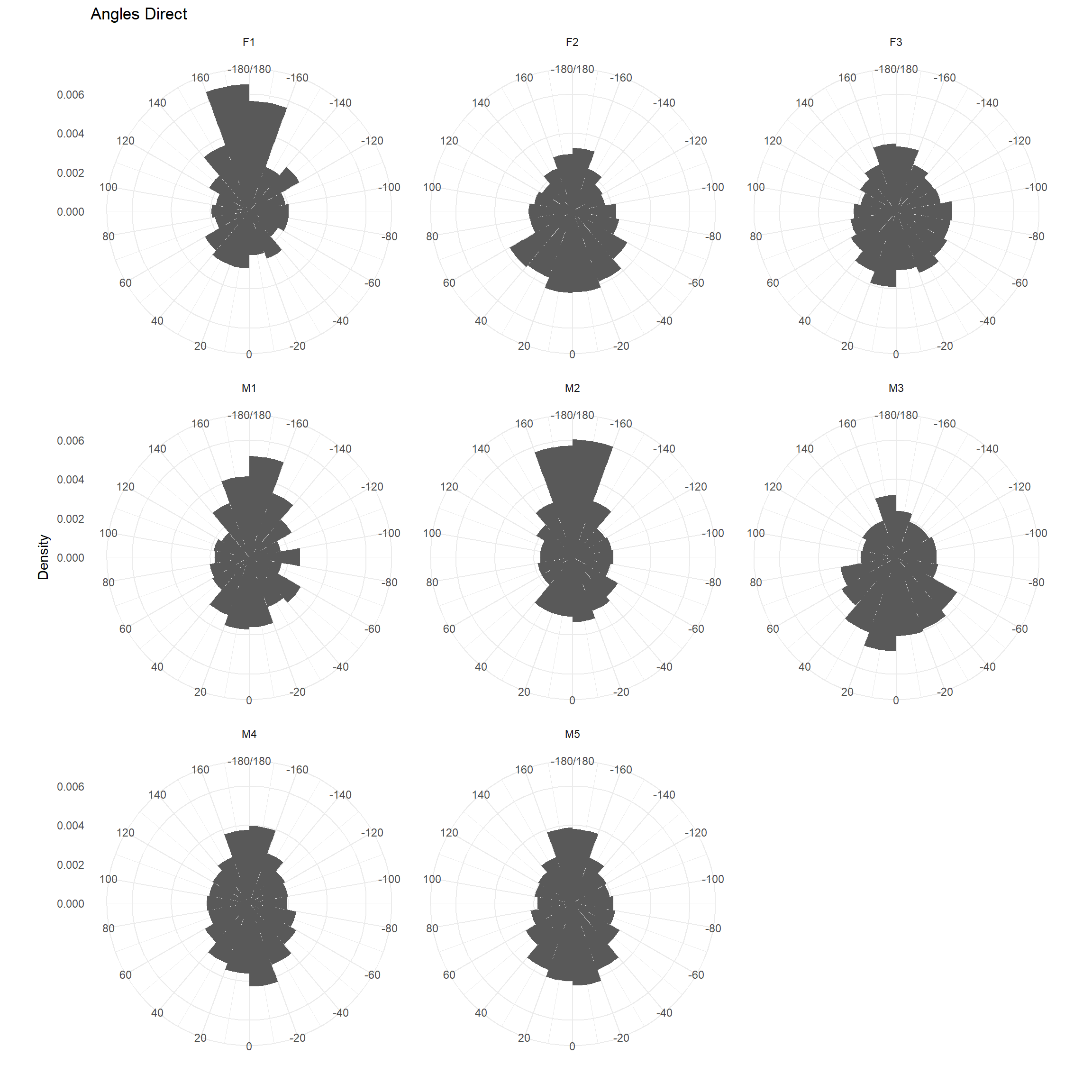### Turning angles as histograms ggplot(trk, aes(x = dir_rel)) + geom_histogram(breaks = seq(-180,180, by=20))+ theme_minimal() + scale_fill_brewer() + ylab("Count") + ggtitle("Angles Relative") + scale_x_continuous("", limits = c(-180, 180), breaks = seq(-180, 180, by=20), labels = seq(-180, 180, by=20))+facet_wrap(~id, scales="free") ## Warning: Removed 16 rows containing non-finite values (stat_bin).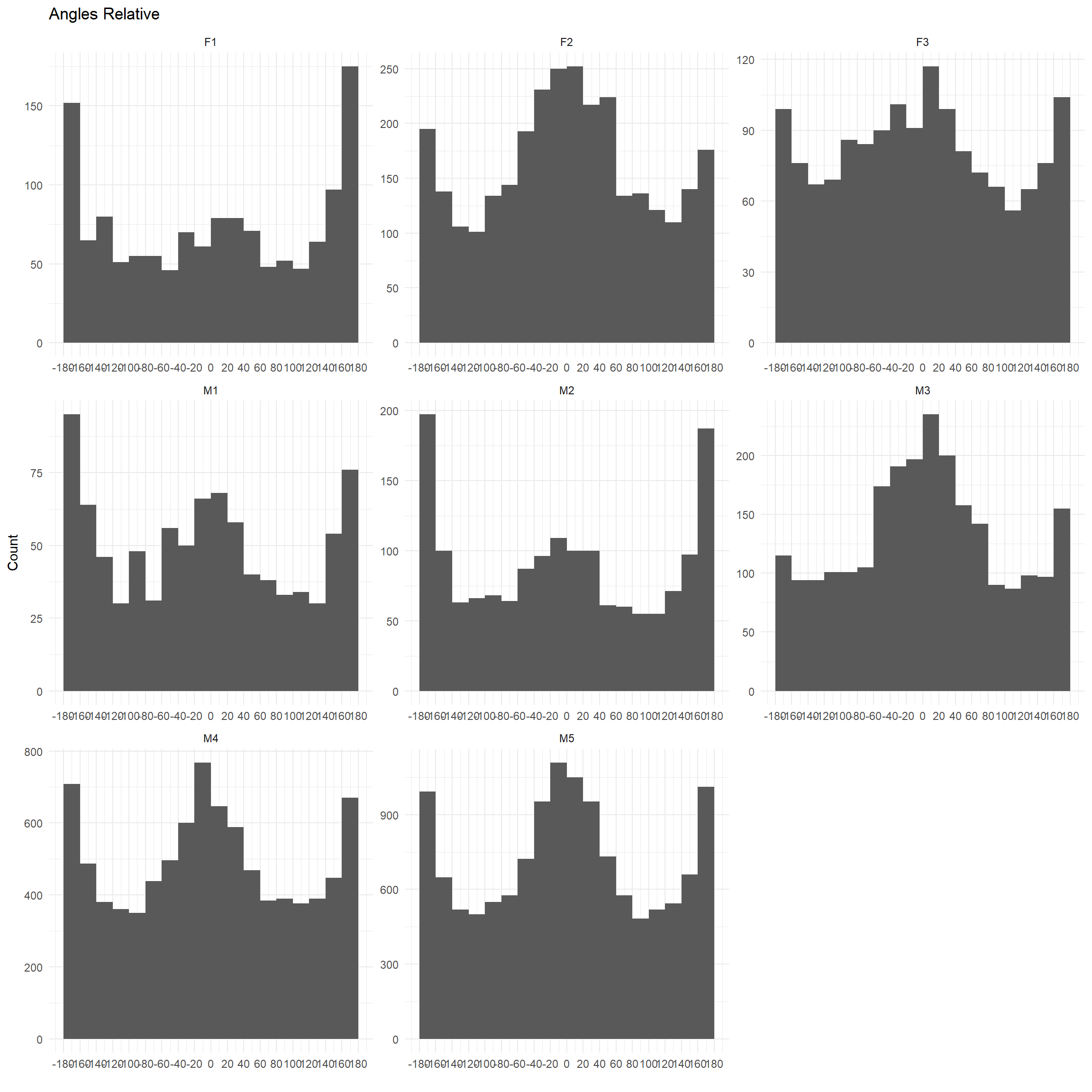### Net-squared displacement over time for each individual ggplot(trk, aes(x = t_, y=nsd_)) + geom_point()+ facet_wrap(~id, scales="free")## Explore movement characteristics by (day/night, hour, month) ### step length distribution by day/night ggplot(trk, aes(x = tod_, y = log(sl))) + geom_boxplot()+geom_smooth()+facet_wrap(~id)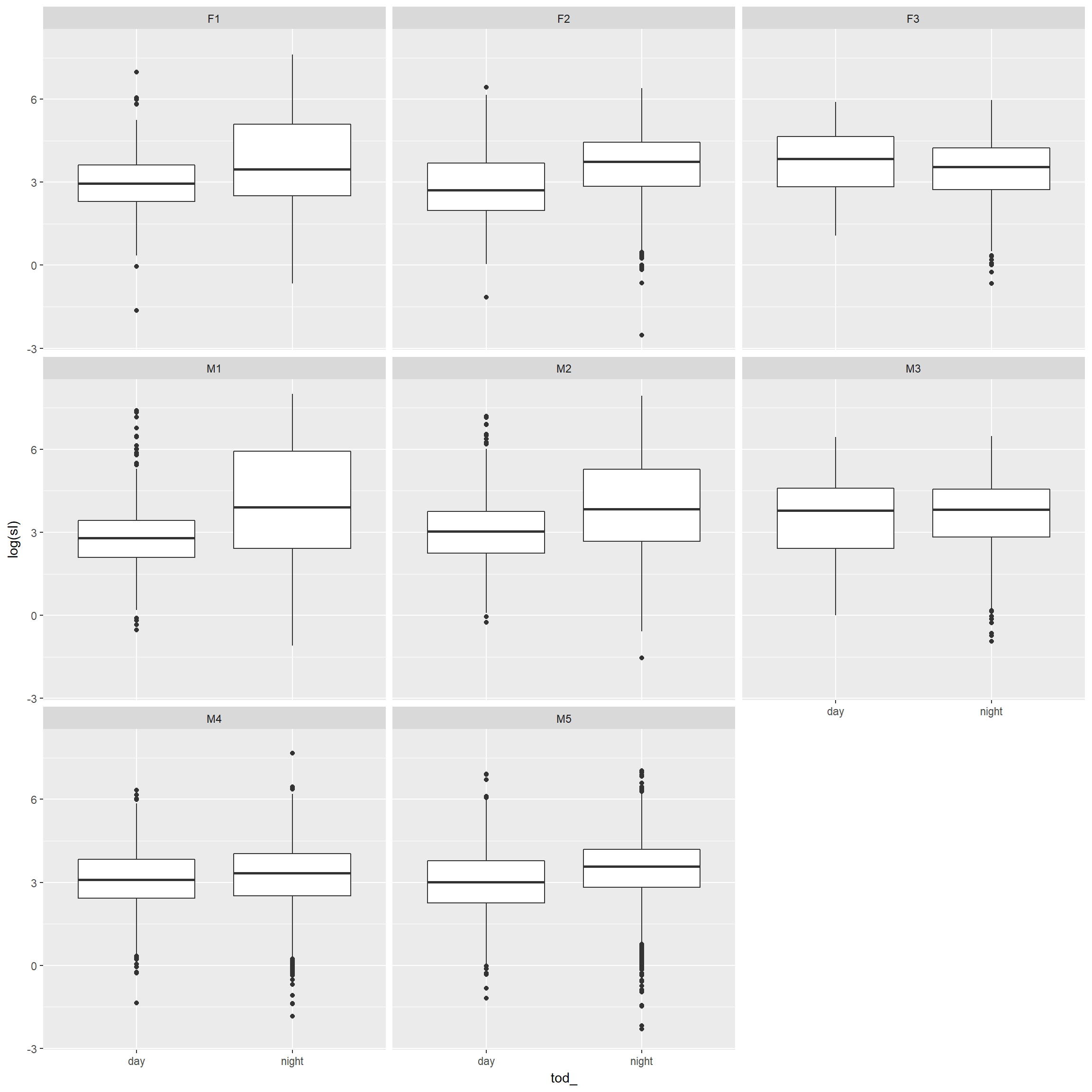### Space use (MCP or KDE) by week, month, and year Note: this code will only work for your critters if you have multiple observations for each combination of (month, year). If you don’t have many observations, you could try: nest(-id, -year) and unnest(id,year) mcps.week<-trk %>% nest(-id,-year, -month, -week) %>% mutate(mcparea = map(data, ~hr_mcp(., levels = c(0.95)) %>% hr_area)) %>% select(id, year, month, week, mcparea) %>% unnest() ggplot(mcps.week, aes(x = week, y = area, colour=as.factor(year))) + geom_point()+ geom_smooth()+ facet_wrap(~id, scales="free")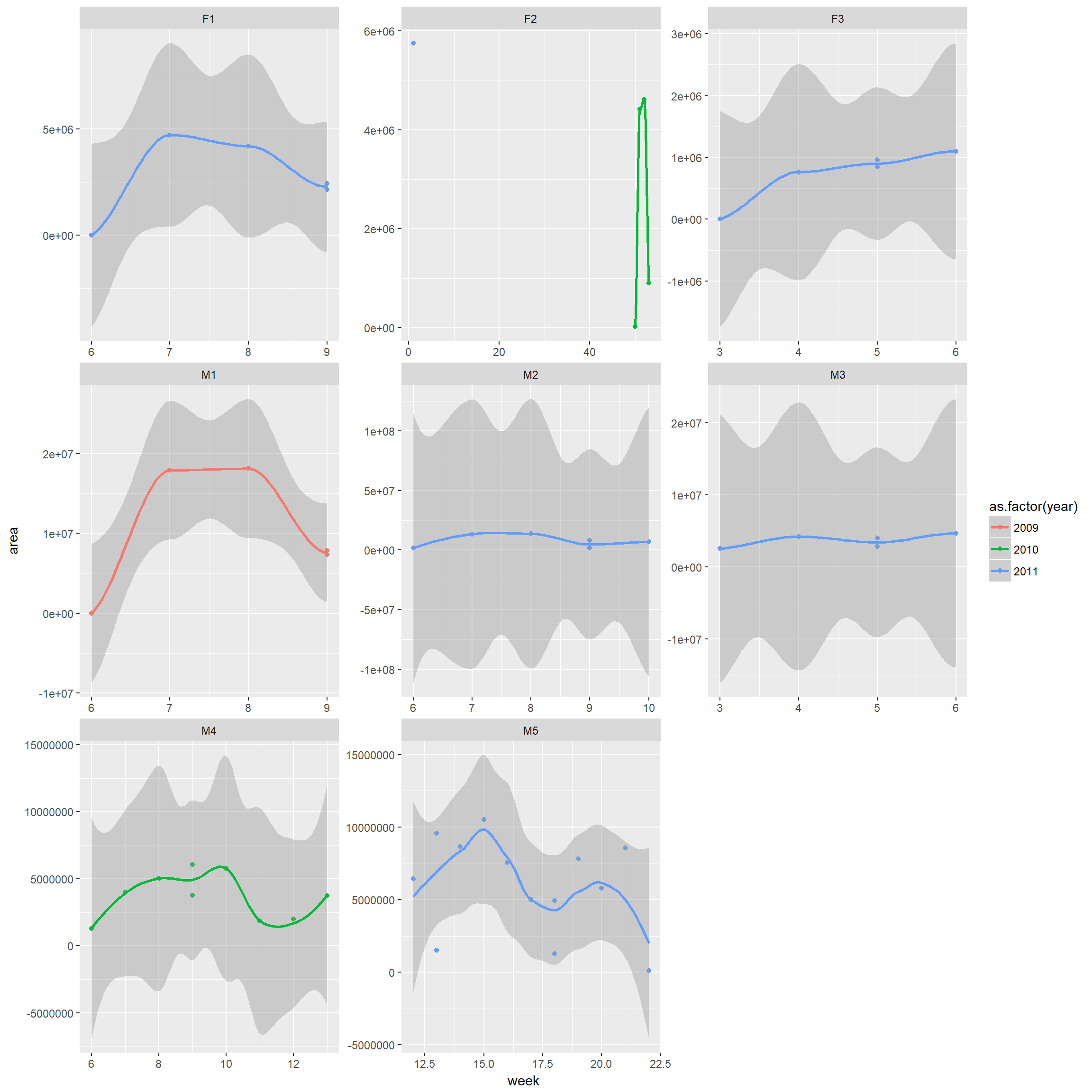Same for KDE kde.week<-trk %>% nest(-id,-year, -month, -week) %>% mutate(kdearea = map(data, ~hr_kde(., levels=c(0.95)) %>% hr_area)) %>% select(id, year, month, week, kdearea) %>% unnest() ggplot(kde.week, aes(x = week, y = kdearea, colour=as.factor(year))) + geom_point()+ geom_smooth()+ facet_wrap(~id, scales="free")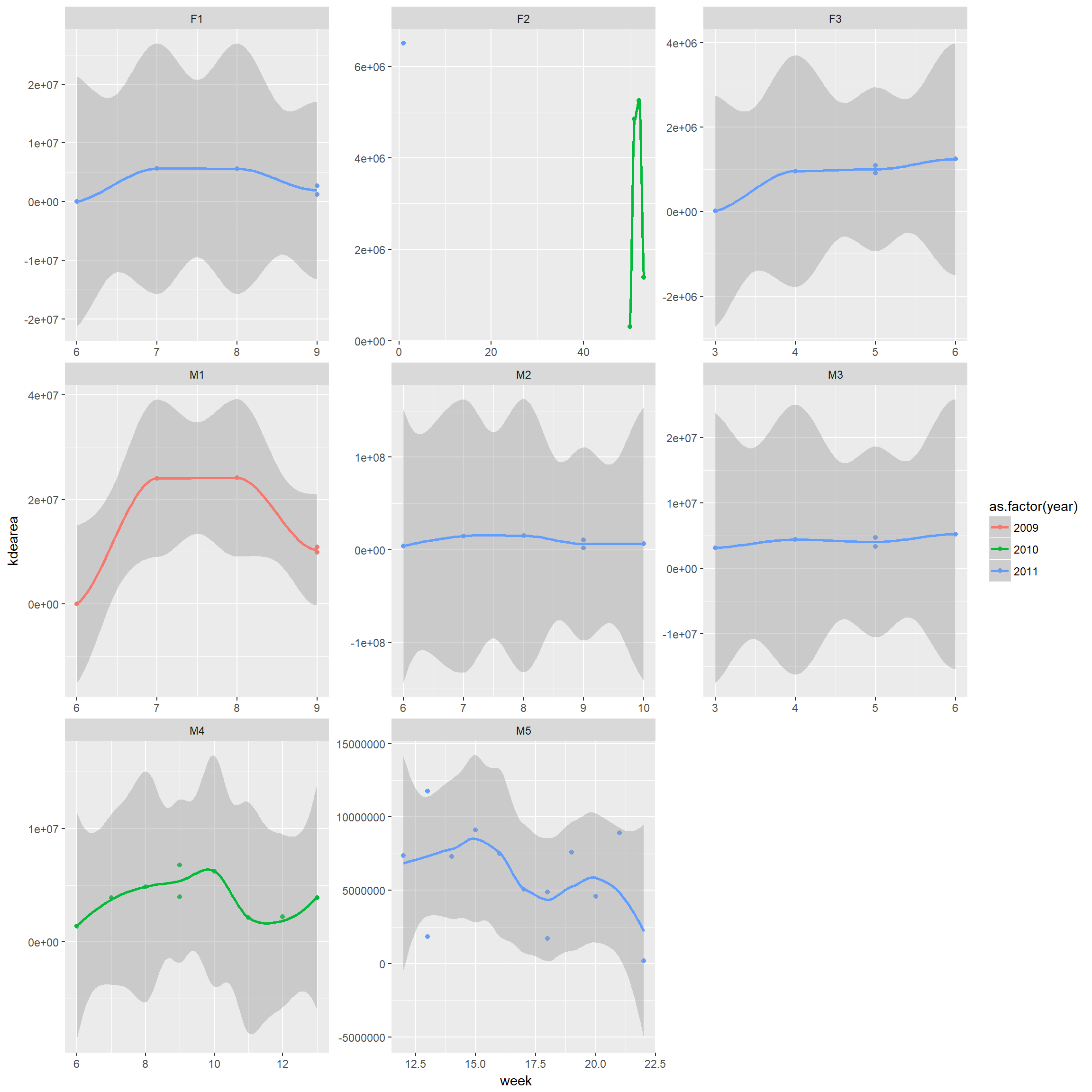## RSF prep Generate random points within MCPs for a single individual using amt functions. Notes: • It is common to generate points randomly, but other options are possible. • In particular, it can beneficial to generate a systematically placed sample • Samples can also be generated using the spsample function in the sp library or using a GIS (note: amt uses the spsample function within its function random_points) • Other home range polygons could be used (e.g., kernel density, local convex hull etc.) #### Random points: illustrate for 1 individual trk %>% filter(id=="F1") %>% random_points(.,factor = 20) %>% plot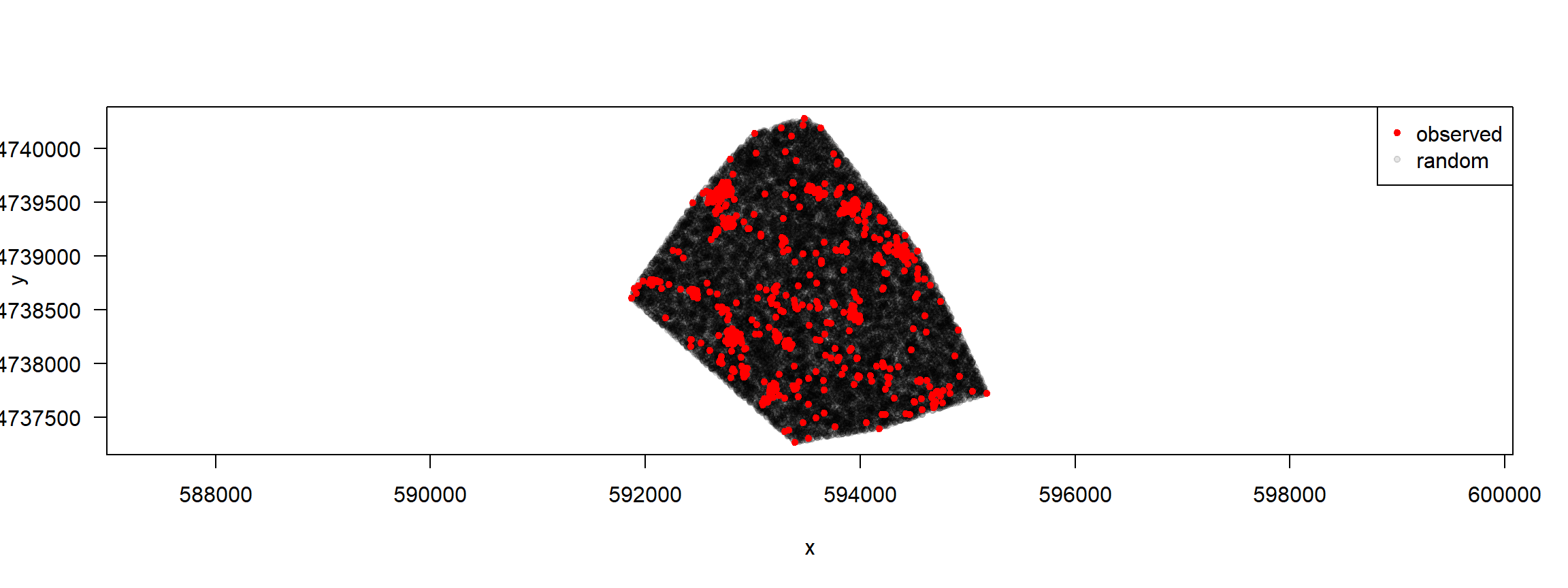Illustrate systematic points (to do this, we need to create the mcp first) trk%>%filter(id=="F1") %>% random_points(., factor = 20, type="regular") %>% plot()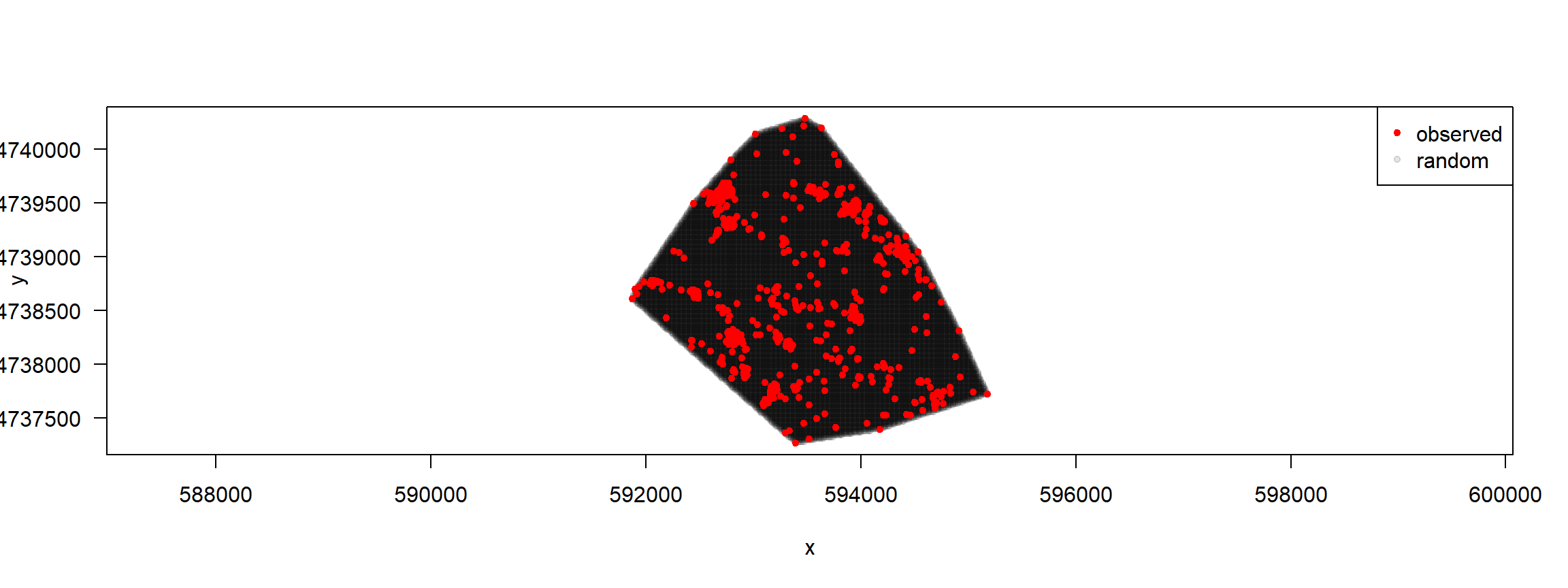Now, lets generate points for all individuals. We can do this efficiently by making use of pipes (%>%),nested data frames, and then by adding a new column – a list-column – to trks avail.pts <- trk %>% nest(-id) %>% mutate(rnd_pts = map(data, ~ random_points(., factor = 20, type="regular"))) %>% select(id, rnd_pts) %>% # you dont want to have the original point twice, hence drop data unnest() Or, we could do this using a loop (commented out, below) #avail.pts<-NULL #uid<-unique(trk$id) # individual identifiers
#luid<-length(uid) # number of unique individuals
#for(i in 1:luid){
# random_points will generate random points within mcp
# Add on the individual id and combine all data
#  temp<-cbind(id=uid[i],trk%>%filter(id==uid[i])%>%random_points)
#  avail.pts<-rbind(avail.pts, temp)
#}
#avail.pts<-as_tibble(avail.pts)
#avail.pts

## Write out data for further annotating

Need to rename variables so everything is in the format Movebank requires for annotation of generic time-location records (see https://www.movebank.org/node/6608#envdata_generic_request). This means, we need the following variables:

• location-lat (perhaps with addition of Easting/Northing in UTMs)
• location-long (perhaps with addition of Easting/Northing in UTMs)
• timestamp (in Movebank format)

Need to project to lat/long, while also keeping lat/long. Then rename variables and write out the data sets.

avail <- SpatialPointsDataFrame(avail.pts[,c("x_","y_")], avail.pts,
proj4string=CRS("+proj=utm +zone=18N +datum=WGS84"))
avail.df <- data.frame(spTransform(avail, CRS("+proj=longlat +datum=WGS84")))[,1:6]
names(avail.df)<-c("idr", "case_", "utm.easting", "utm.northing", "location-long", "location-lat")

Check to make sure everything looks right

test<-subset(avail.df, case_==TRUE)
test %>% select('location-lat', 'location-long', utm.easting, utm.northing) %>%
summarise_all(mean)
##   location-lat location-long utm.easting utm.northing
## 1     42.80687     -73.69359    606812.2      4740221
fisher.dat %>% summarize(meanloc.lat=mean(location_lat),
meanloc.long=mean(location_long))
##   meanloc.lat meanloc.long
## 1    42.80687    -73.69359

Add a timestamp to annotate these data with environmental covariates in Movebank using Env-DATA (https://www.movebank.org/node/6607). Here we just use the first timestamp, however meaningful timestamps are needed if annotating variables that vary in time.

avail.df$timestamp<-fisher.dat$timestamp

These points then need to be annotated prior to fitting rsfs. Let’s write out 2 files:

• FisherRSF2018.csv will contain all points and identifying information.
• FisherRSFannotate.csv will contain only the columns used to create the annotation.

The latter file will take up less space, making it easier to annotate (and also possible to upload to github)

write.csv(avail.df, file="data/FisherRSF2018.csv", row.names = FALSE)

avail.df<-avail.df %>% select("timestamp", "location-long", "location-lat")
write.csv(avail.df, file="data/FisherRSFannotate.csv", row.names = FALSE)

## SSF prep

SSFs assume that data have been collected at regular time intervals. We can use the track_resample function to regularize the trajectory so that all points are located within some tolerence of each other in time. To figure out a meaningful tolerance range, we should calculate time differences between locations & look at as a function of individual.

(timestats<-trk %>% nest(-id) %>% mutate(sr = map(data, summarize_sampling_rate)) %>%
select(id, sr) %>% unnest)
## # A tibble: 8 x 10
##   id        min    q1 median  mean    q3   max    sd     n unit
##   <fct>   <dbl> <dbl>  <dbl> <dbl> <dbl> <dbl> <dbl> <int> <chr>
## 1 M1    13.3    14.7   15.0  32.7  15.5   977. 104.    918 min
## 2 M4     0.1000  1.93   2.03  8.04  2.57 1209.  44.0  8957 min
## 3 F2     0.400   1.97   2.03  8.93  3.98 1080.  48.1  3003 min
## 4 M3     0.417   1.97   2.17 13.3   5.42 2889.  90.2  2435 min
## 5 F3     0.417   1.97   2.07 15.7   4.08 2167. 104.   1500 min
## 6 M2     1.35    9.78  10.0  21.7  10.3  2111.  87.2  1637 min
## 7 F1     3.92    9.80  10.0  20.7  10.3  1650.  95.2  1348 min
## 8 M5     0.250   1.93   2.02  7.41  2.37  976.  25.0 13098 min

Time intervals range from every 2 to 15 minutes on average, depending on the individual. Lets add on the time difference to each obs.

trk<-trk %>% group_by(id) %>% mutate(dt_ = t_ - lag(t_, default = NA))

Let’s illustrate track regularization with ID = F2. Let’s go from every 2 minutes to every 10.

tempF2<-trk %>% filter(id=="F2") %>% track_resample(rate=minutes(10), tolerance=minutes(2))
tempF2 %>% select(id, x_, y_, t_, burst_)
## # A tibble: 1,010 x 5
## # Groups:   id 
##    id         x_       y_ t_                      burst_
##  * <fct>   <dbl>    <dbl> <dttm>                   <dbl>
##  1 F2    590028. 4733219. 2010-12-16 16:45:30.999      1
##  2 F2    590486. 4733344. 2010-12-16 21:21:13.999      2
##  3 F2    591220. 4733065. 2010-12-16 21:32:34.000      2
##  4 F2    591215. 4733037. 2010-12-17 08:57:00.999      3
##  5 F2    591172. 4733057. 2010-12-17 09:05:14.000      3
##  6 F2    591075. 4733081. 2010-12-17 09:16:29.000      3
##  7 F2    591024. 4733025. 2010-12-17 09:24:53.000      3
##  8 F2    590793. 4732994. 2010-12-17 09:37:24.000      4
##  9 F2    590838. 4733077. 2010-12-18 01:08:42.997      5
## 10 F2    590658. 4733176. 2010-12-18 01:24:34.000      6
## # ... with 1,000 more rows

Now loop over individuals and do the following:

• Regularize trajectories using an appropriate time window (see e.g., below)
• calculate new dt values
• Create bursts using individual-specific time intervals
• Generate random steps within each burst

The random steps are generated using the following approach:

1. Fit a gamma distribution to step lenghts
2. Fit a von mises distribution to turn angles
3. Use these distribution to draw new turns and step lengths, form new simulated steps and generate random x,y values.
ssfdat<-NULL
temptrk<-with(trk, track(x=x_, y=y_, t=t_, id=id))
uid<-unique(trk$id) # individual identifiers luid<-length(uid) # number of unique individuals for(i in 1:luid){ # Subset individuals & regularize track temp<-temptrk%>% filter(id==uid[i]) %>% track_resample(rate=minutes(round(timestats$median[i])),
tolerance=minutes(max(10,round(timestats$median[i]/5)))) # Get rid of any bursts without at least 2 points temp<-filter_min_n_burst(temp, 2) # burst steps stepstemp<-steps_by_burst(temp) # create random steps using fitted gamma and von mises distributions and append rnd_stps <- stepstemp %>% random_steps(n = 15) # append id rnd_stps<-rnd_stps%>%mutate(id=uid[i]) # append new data to data from other individuals ssfdat<-rbind(rnd_stps, ssfdat) } ssfdat<-as_tibble(ssfdat) ssfdat ## # A tibble: 470,000 x 13 ## burst_ step_id_ case_ x1_ y1_ x2_ y2_ t1_ t2_ dt_ sl_ ta_ id ## <dbl> <int> <lgl> <dbl> <dbl> <dbl> <dbl> <dttm> <dttm> <time> <dbl> <dbl> <fct> ## 1 1 1 TRUE 630498. 4742879. 630569. 4742823. 2011-03-22 00:02:12.997 2011-03-22 00:04:10.997 118 90.4 -62.6 M5 ## 2 1 1 FALSE 630498. 4742879. 630531. 4742824. 2011-03-22 00:02:12.997 2011-03-22 00:04:10.997 118 33.3 -113. M5 ## 3 1 1 FALSE 630498. 4742879. 630509. 4742929. 2011-03-22 00:02:12.997 2011-03-22 00:04:10.997 118 107. -130. M5 ## 4 1 1 FALSE 630498. 4742879. 630500. 4742826. 2011-03-22 00:02:12.997 2011-03-22 00:04:10.997 118 3.90 -125. M5 ## 5 1 1 FALSE 630498. 4742879. 630506. 4742823. 2011-03-22 00:02:12.997 2011-03-22 00:04:10.997 118 7.73 50.2 M5 ## 6 1 1 FALSE 630498. 4742879. 630473. 4742855. 2011-03-22 00:02:12.997 2011-03-22 00:04:10.997 118 40.4 77.6 M5 ## 7 1 1 FALSE 630498. 4742879. 630526. 4742862. 2011-03-22 00:02:12.997 2011-03-22 00:04:10.997 118 48.2 -150. M5 ## 8 1 1 FALSE 630498. 4742879. 630574. 4742643. 2011-03-22 00:02:12.997 2011-03-22 00:04:10.997 118 195. 143. M5 ## 9 1 1 FALSE 630498. 4742879. 630534. 4742723. 2011-03-22 00:02:12.997 2011-03-22 00:04:10.997 118 107. 80.5 M5 ## 10 1 1 FALSE 630498. 4742879. 630495. 4742822. 2011-03-22 00:02:12.997 2011-03-22 00:04:10.997 118 3.41 28.8 M5 ## # ... with 469,990 more rows Now, lets plot the data for random and matched points ggplot(ssfdat, aes(x2_, y2_, color=case_))+geom_point()+facet_wrap(~id, scales="free")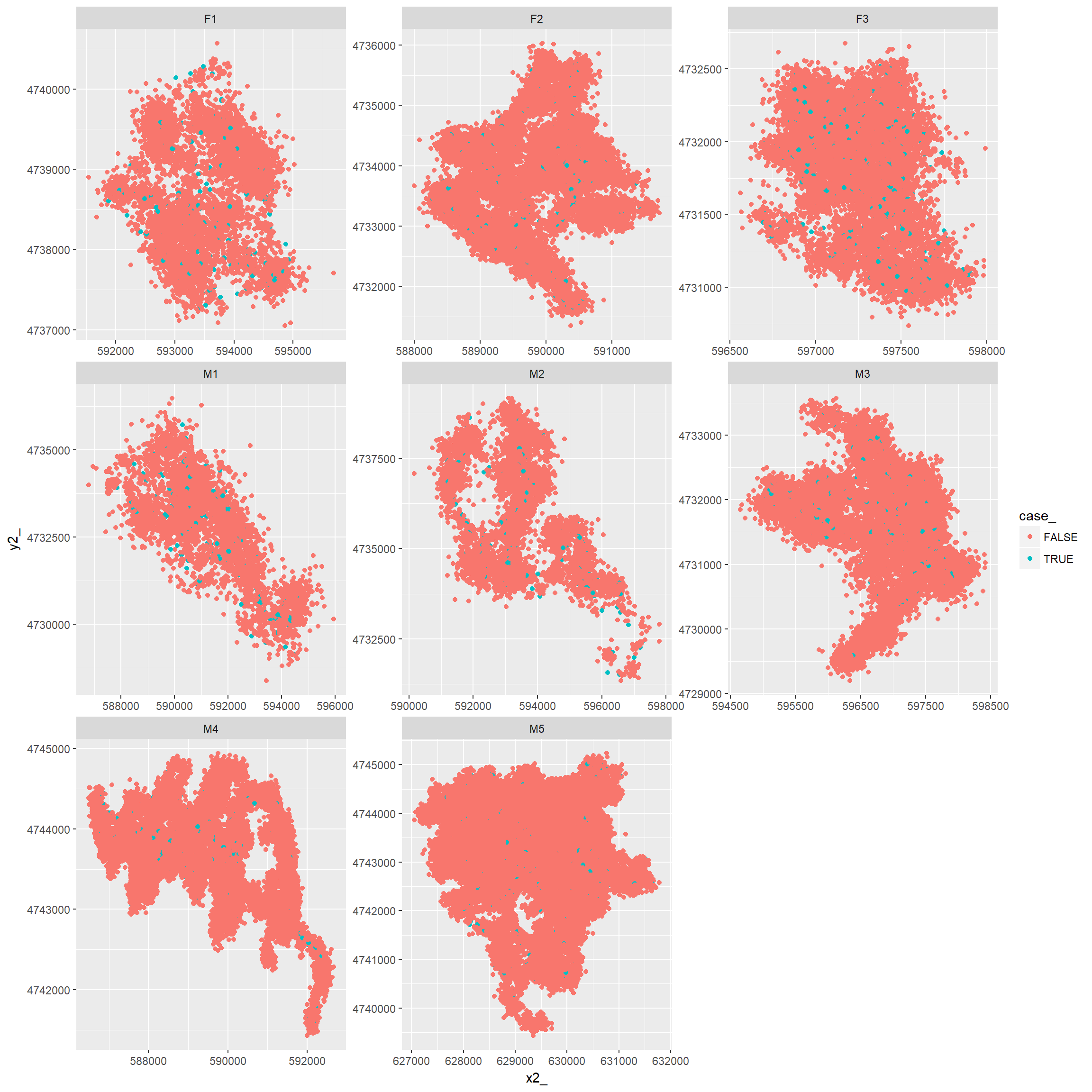Relabel as utms ssfdat$utm.easting<-ssfdat$x2_ ssfdat$utm.northing<-ssfdat$y2_ ## Write out data for further annotating Need to rename variables so everything is in the format Movebank requires for annotation of generic time-location records (see https://www.movebank.org/node/6608#envdata_generic_request). This means, we need the following variables: • location-lat (perhaps with addition of Easting/Northing in UTMs) • location-long (perhaps with addition of Easting/Northing in UTMs) • timestamp (in Movebank format) Need to project to lat/long, while also keeping lat/long. Then rename variables and write out the data sets. With the SSFs, we have the extra complication of having a time and location at both the start and end of the step. For the time being, we will assume we want to annotate variables at the end of the step but use the starting point of the step as the timestamp. You could also calculate the midpoint of the timestep like this: data$timestamp.midpoint <- begintime + (endtime-begintime)/2

ssfdat2 <- SpatialPointsDataFrame(ssfdat[,c("x2_","y2_")], ssfdat,
proj4string=CRS("+proj=utm +zone=18N +datum=WGS84"))
ssf.df <- data.frame(spTransform(ssfdat2, CRS("+proj=longlat +datum=WGS84")))
names(ssf.df)[c(13,16,17)] <-c("individual.local.identifier", "location-long", "location-lat")
ssf.df$timestamp<-ssf.df$t1_
ssf.df %>% select('location-lat', utm.easting, x1_, x2_, y1_, y2_, 'location-long', utm.northing) %>% head
##   location-lat utm.easting      x1_      x2_     y1_     y2_ location-long utm.northing
## 1     42.82684    630568.9 630497.9 630568.9 4742879 4742823     -73.40259      4742823
## 2     42.82685    630531.2 630497.9 630531.2 4742879 4742824     -73.40305      4742824
## 3     42.82780    630509.4 630497.9 630509.4 4742879 4742929     -73.40329      4742929
## 4     42.82688    630500.4 630497.9 630500.4 4742879 4742826     -73.40342      4742826
## 5     42.82685    630505.6 630497.9 630505.6 4742879 4742823     -73.40336      4742823
## 6     42.82714    630472.7 630497.9 630472.7 4742879 4742855     -73.40375      4742855

These points then need to be annotated prior to fitting ssfs. Let’s write out 2 files:

• FisherSSF2018.csv will contain all points and identifying information.
• FisherSSFannotate.csv will contain only the columns used to create the annotation.

The latter file will take up less space, making it easier to annotate (and also possible to upload to github)

write.csv(ssf.df, file="data/AllStepsFisher2018.csv", row.names=FALSE)
ssf.df<-ssf.df %>% select("timestamp", "location-long", "location-lat")
write.csv(ssf.df, file="data/FisherSSFannotate.csv", row.names = FALSE)

## Using nested data frames

This works if all animals are sampled at a constant sampling rate. Again, you need to create a new column (using mutate) where you save the random steps to Not all animals have a 15 min sampling rate, so we might drop the first animal that has a 15 minute sampling rate.

#trk %>% nest(-id) %>% mutate(sr = map(.\$data, summarize_sampling_rate)) %>%
#  select(id, sr) %>% unnest()

# Then, we could avoid a loop with:
#ssfdat <- trk %>% filter(id != "M1") %>% nest(-id) %>%
#  mutate(ssf = map(data, function(d) {
#    d %>%
#      track_resample(rate = minutes(10), tolerance = minutes(2)) %>%
#      filter_min_n_burst(min_n = 3) %>%
#      steps_by_burst() %>% random_steps()
#  })) %>% select(id, ssf) %>% unnest()